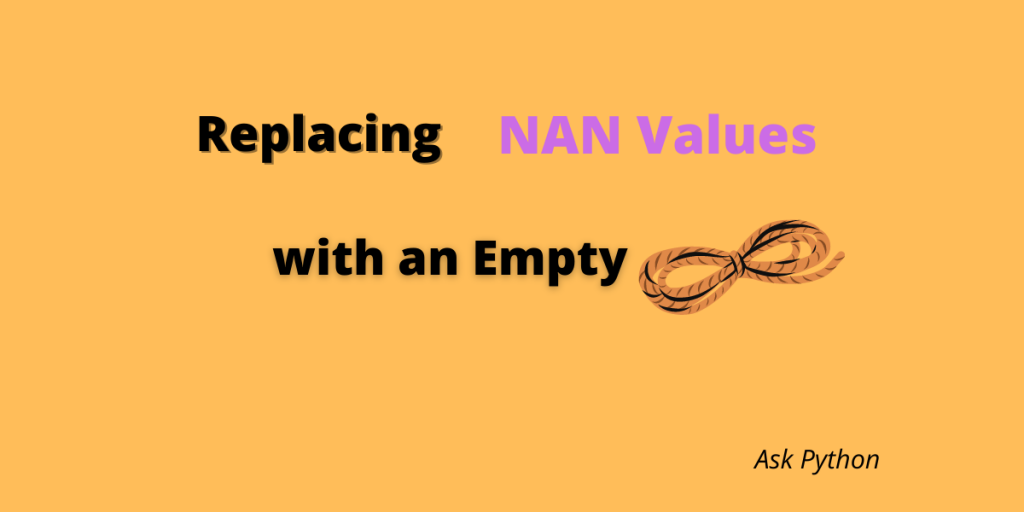# How to Replace NAN Values in Pandas with an Empty String?An important part of the data analysis process is getting rid of the NAN values. In this article, how to replace NAN values in one column or multiple columns with an empty string. Let’s get started!

Also read: Understanding NaN in Numpy and Pandas

We will start by creating a DataFrame. Let’s create a DataFrame with the data of marks scored by the students in different subjects. The columns are “Name,” “Score,” and “Age.”

```import pandas as pd
import numpy as np
scoresheet = {
'Name' :['Linda','Tommy','Justin','Gary',np.nan],
'Score':['60',np.nan,'50','70','80'],
'Age':['18','19',np.nan,'20','22'],
}
df = pd.DataFrame(scoresheet)
print(df)
```

Output

```     Name     Score      Age
0   Linda    60            18
1   Tommy NaN        19
2  Justin    50           NaN
3    Gary    70           20
4     NaN    80           22
```

## 4 Methods to replace NAN with an empty string

Let’s now learn how to replace NaN values with empty strings across an entire dataframe in Pandas

### 1. Using df.replace(np.nan,’  ‘, regex=true) method

This method is used to replace all NAN values in a DataFrame with an empty string.

```df2 = df.replace(np.nan, '', regex=True)
print(df2)
```

Output

```     Name       Score     Age
0   Linda         60          18
1   Tommy                     19
2  Justin          50
3    Gary         70            20
4                    80            22
```

### 2. Using df [[‘column1′,’column2’]] = df [[‘column1′,’column2’]] . fillna(”) method

In this method, we will only replace the NAN values in the columns that are specified.

```df2 = df[['Age','Score' ]] = df[['Age','Score' ]].fillna('')
print(df2)
```

Output

``` Age Score
0  18    60
1  19
2          50
3  20    70
4  22    80
```

### 3. Using the fillna() method

The fillna() method can be used to replace all the NAN values in a DataFrame.

```df2 = df.fillna("")
print(df2)
```

Output

```     Name      Score    Age
0   Linda        60         18
1   Tommy                   19
2  Justin         50
3    Gary         70         20
4                    80          22
```

### 4. Using the fillna() method on a specific column

In this method, we will use the fillna() method for a specific column in the DataFrame.

```df2 = df.Age.fillna('')
print(df2)
```

Output

```0    18
1    19
2
3    20
4    22
Name: Age, dtype: object
```

## Conclusion

In summary, we looked at the various different methods of filling a NAN value in a DataFrame with an empty string. It is a very important step in data analysis and it is necessary that you know how to get rid of the NAN values.